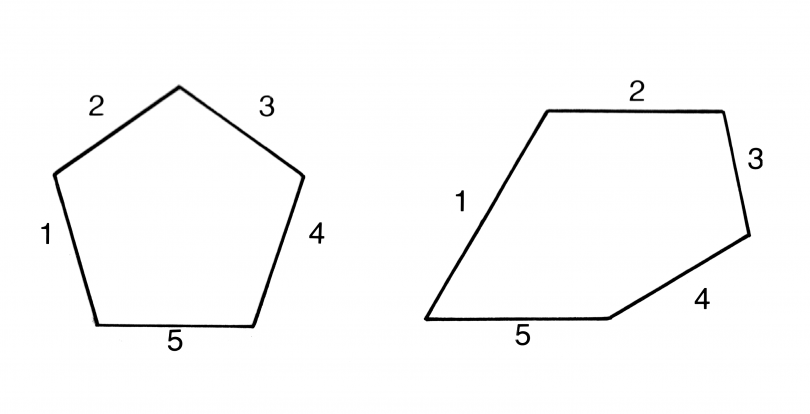Education

# Tips to Remember the Formulas in MathematicsWritten by
Learning mathematical formulas is quite tough in the beginning. Mathematics is full of formulas and equations. But once you get to know the simple and basic techniques to learn the formulas, you will find a positive attitude towards mathematics. Some tips are given below to remember the formulas easily.

## Identify the Problem Area

To remember the formulas in mathematics first, you have to analyse the weaker areas. Once you realise your problem area, it helps you to learn in a better way. One of the best technique to remember the formulas is to create a story for a required situation. Create a story sequence to remember the formulas in a particular order.

## Be Creative

Be creative with whatever you are learning. Always visualise the concepts when you are learning. Imagining the ideas helps to remember the concepts at any time. Always try to understand the concepts using simple examples. For example to learn the formulas like area and volume of different shapes in maths, try to relate different shapes with the objects that you are using in the daily life routine.

## Learn and Practice

Always learn the formulas in a specific pattern. You can quickly learn it by forming simple games and riddles. A traditional way to remember the formulas is to read it again and again and also writing down the formulas many times. Learn to derive each formula. Once you learn to derive the formula from the root concepts, you will become so familiar with the formulas, that it will help retain in your brain for a long time. Practice the formulas as many times as possible and learn the formulas for a question to find the solution.

## Don’t Mug Up

The formulas that you are learning in academic life not only helps to score good marks. It is applied in each and every perspective of life. Instead of mugging up the formulas, understand the concept. Then try to apply it practically to solve the problem for the given circumstances. In certain situations memorizing the formulas doesn’t work. Learning the concepts matters a lot. If you want to find the lcm and hcf of a number, you should know the concept to find the factors of a number either by using a factorization method or division method.

To know more tips and tricks to crack the examination, subscribe to BYJU’S YouTube channel and also watch interesting videos for more such information.